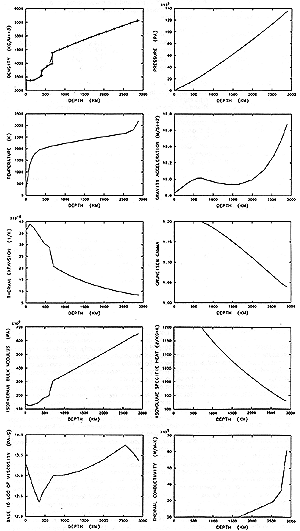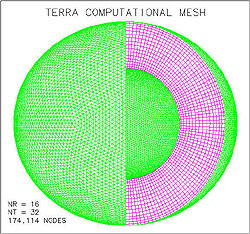COMPUTER MODELING OF THE LARGE-SCALE TECTONICS ASSOCIATED WITH THE GENESIS FLOOD
Figures
Click on any of the figures below to get a larger image.
 Figure 1. Radial profiles of density, pressure, temperature, gravitational acceleration, thermal expansivity, Grueneisen gamma, isothermal bulk modulus, isovolume specific heat, base 10 log of dynamic viscosity, and thermal conductivity for the earth reference state. Crosses and dotted line on density profile is the density for the Preliminary Reference Earth Model (PREM) of Dweiwonski and Anderson .Figure 2. Computational mesh derived from the regular icosahedron. Mesh has 17 layers of nodes with 10242 nodes per layer.Figure 3. Initial conditions for 3-D calculation. (a) Initial plate boundaries represent present continents mapped to their approximate Pangean locations. Blue regions represent thermal anomaly 400 K colder than reference temperature at a depth of 100km. (b) Cross section through equatorial plane viewed from the north pole displaying deviations from the reference temperature. Temperature perturbations representing incipient subduction have a depth extent of 400 km.
 Figure 4. Temperature (in color), velocities, and continent boundaries at 100 km depth at times of (a) 10 days, (b) 30 days, (c) 50 days, and (d) 70 days. Velocity magnitude is proportional to arrow length. Peak velocities at this depth are (a) 0.37 m/s, (b) 0.59 m/s, (c) 1.04 m/s, and (d) 0.98 m/s.
 Figure 5. Cross sections through the equatorial plane viewed from the north pole showing velocities and temperature deviation from the reference temperature at (a) 10 days, (b) 30 days, (c) 50 days, and (d) 70 days. Range of temperature deviation shown is -500 K to 500 K. Velocity magnitude is proportional to arrow length. Peak velocities are (a) 0.66 m/s, (b) 1.14 m/s, (c) 2.35 m/s, and (d) 2.54 m/s.
 Figure 6. Surface topography in meters relative to local sea level at a time of 30 days. Purple contour indicates sea level. Note that regions near divergent plate margins are elevated and continental areas adjacent to subduction zones are flooded.